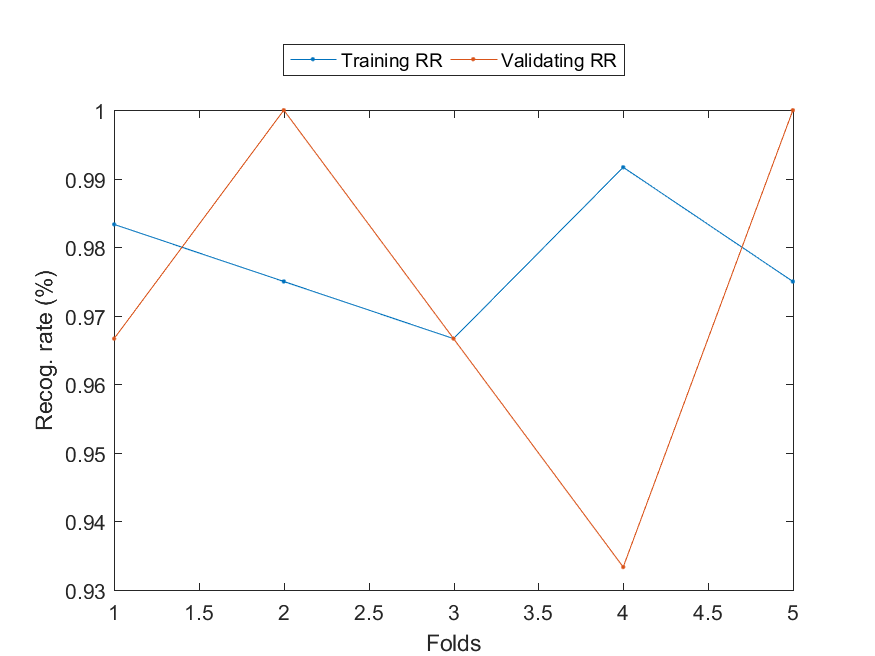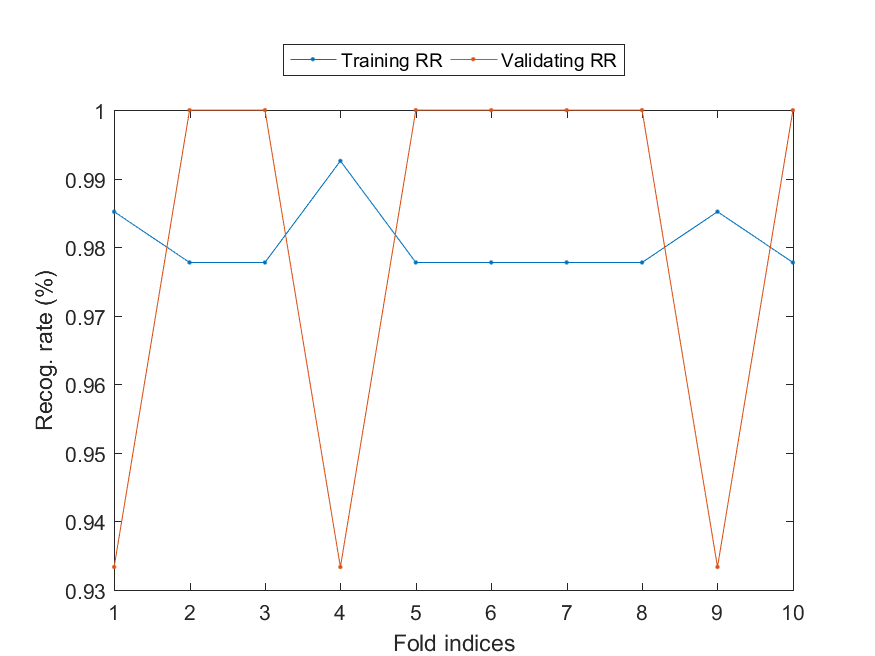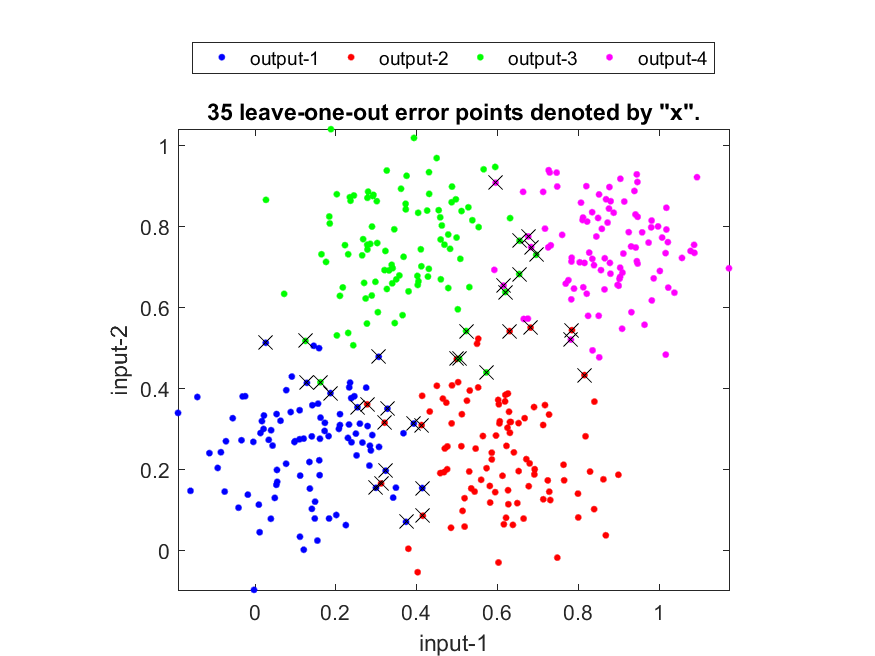6-2 Methods for Recognition Rate Estimate (嚙踝蕭嚙踝蕭嚙踝蕭嚙踝蕭嚙踝蕭嚙踝蕭嚙踝蕭嚙?

[chinese][all]

In this section, we shall introduce the estimation of recognition rates (or error rates) for a classifier that can be constructed by any pattern recognition methods.

If we use the same dataset for both training and test, then the obtained recognition rate is referred to as the inside-test recognition rate or the resubstitution recognition rate. This inside-test result is usually overly optimistical since all data is used for training and the test is also based on the same data. In particular, if we use 1-NNR for our classifier, then the inside-test recognition rate will always be 100%.

Though the inside-test recognition rate is not objective, it can serve as the upper-bound of the true recognition rate. In general, we use the inside-test recognition rate as a first step for examining our classifier. If the inside-test recognition rate is already low, there are two possible reasons:

• The design method for the classifier is not good enough.
• The features of the training set do not have good discrinimative power.

However, if the inside-test recognition rate is high, it does not mean we have reach a reliable classifier. Usually we need to prepare a set of "unseen" data to test the classifier, as explained next.

After a classifier is constructed, usually it will face unseen data for further application. Therefore it is better to prepare a set of "unseen" data for evaluating the recognition rate of the classifier. In practice, we usually divide the available data set into two disjoint part of a training set and a test set. The training set is used for designing the classifier, while the test set is used for evaluating the recognition rate of the classifier. The obtained recognition rate is referred to as the outside-test recognition rate or the holdout recognition rate, with the following characteristics:

• Since the test set is not used for designing the classifier, the obtained recognition rate is more objective.
• Since the available data set is of limited size in the real world, the outside-test recognition rate is a little bit lower than the true recognition rate since a part of the data set is set aside for test.
• The complexity of a classifier is defined as the number of free parameters in the classifier. In general, the inside-test recognition goes up with the complexity of the classifier. On the other hand, the outside-test recognition rate goes up with the complexity of the classifier initially, but then goes down afterwords due to over-training. Usually we select the number of free parameters of a classifier which can optimize the outside-test recognition rate.
• After we set up the complexity of the classifier, we can then use the whole dataset for training. We can expect the true recognition rate of the thus-constructed classifier should be a little bit higher than the optimum outside-test recognition rate mentioned earlier.

We can extend the concept of outside test to have the so-called two-fold cross validation or two-way outside-test recognition rate. Namely, we can divide the data set into part A and B of equal size. In the first run, we use part A as the training set and part B as the test set. In the second run, we reverse the roles of part A and B. The overall recognition rate will be the average of these two outside-test recognition rates.

In two-fold cross validation, the dataset is divided into two equal-size parts, which lead to slight lower outside-test recognition rates since each classifier can only use 50% of the dataset. In order to estimate the recognition rate better, we can have m-fold cross validation in which the dataset S is divided into m sets of about equal size, S1, S2, ..., Sm, with the following characteristics:

• S = S1∪S2∪...∪Sm
• |S1| = |S2| = ... = |Sm|
• Si∩Sj = φ (empty set) whenever i≠j.
• The class distribution of each Sj, i=1 to m, should be as close as possible to that of the original dataset S.

Then we estimate the recognition according to the following steps:

1. Use Si as the test set, while all the other data S-Si as the training set to design a classifier. Test the classifier using Si to obtain the outside-test recognition rate.
2. Repeat the above step for each of Si, i = 1 to m. Compute the overall average outside-test recognition rate.

The following example demonstrate the use of 5-fold cross validation on the IRIS dataset.

Example 1: rreViaCv01.mdataSet=prData('iris'); m=5; cvOpt=cvDataGen('defaultOpt'); cvOpt.foldNum=m; cvOpt.cvDataType='full'; cvData=cvDataGen(dataSet, cvOpt); foldNum=length(cvData); % Actual no. of folds for i=1:foldNum [qcPrm, logProb1, tRr(i)]=qcTrain(cvData(i).TS); tSize(i)=length(cvData(i).TS.output); [computedClass, logProb2, vRr(i)]=qcEval(cvData(i).VS, qcPrm); vSize(i)=length(cvData(i).VS.output); end tRrAll=dot(tRr, tSize)/sum(tSize); vRrAll=dot(vRr, vSize)/sum(vSize); plot(1:foldNum, tRr, '.-', 1:foldNum, vRr, '.-'); xlabel('Folds'); ylabel('Recog. rate (%)'); legend('Training RR', 'Validating RR', 'location', 'northOutside', 'orientation', 'horizontal'); fprintf('Training RR=%.2f%%, Validating RR=%.2f%%\n', tRrAll*100, vRrAll*100); Training RR=97.83%, Validating RR=97.33%Since this type of performance evaluation using cross-validation is used often, we have created a function to serve this purpose, as shown in the next example where 10-fold cross-validation is applied to IRIS dataset:

Example 2: perfCv4qc01.mDS=prData('iris'); showPlot=1; foldNum=10; classifier='qc'; [vRrAll, tRrAll]=perfCv(DS, classifier, [], foldNum, showPlot); fprintf('Training RR=%.2f%%, Validating RR=%.2f%%\n', tRrAll*100, vRrAll*100); Training RR=98.07%, Validating RR=98.00%A larger m will require more computation for constructing m classifiers. In practice, we select the value of m based on the size of the data set and the time needed to construct a specific classifier. In particular,

• When m is equal to 2, we have the simple case of two-fold cross validation.
• When m is equal to n (the size of the dataset), we have the leave-one-out method to be explained next.

Leave-one-out method is also known as the jackknife procedure, which the most objective method for recognition rate estimate since almost all the data (except one entry) is used for constructing the classifier. It involves the following steps:

Use xi (the i-th entry in the dataset) as the test set, while all the other data as the training set to design a classifier. Test the classifier using xi to obtain the outside-test recognition rate (which is either 0% or 100%).
1. Repeat the above step for each of xi, i = 1 to n. Compute the overall average outside-test recognition rate.

The obtained recognition rate is known as the leave-one-out (LOO for short) recognition rate. The leave-one-out method has the following characteristics:

• Each classifier uses almost all the dataset (except one entry), therefore the outside-test recognition rate should be able to approach the true recognition rate closely.
• For classifiers that require massive computation in the design stage (such as artificial neural networks, Gaussian mixture models), the leave-one-out method is impractical for a moderate dataset.
• Since the leave-one-out method require a lot more computation, usually we only choose a simple classifier such as KNNC for estimating the LOO recognition rate. The obtained LOO recognition rate can help us have a rough idea of the discriminating power of the features in the dataset.

In the following example, we use the function knncLoo.m to find the LOO recognition rates based 1-NNR. Each misclassified data point is labeled with a cross for easy visual inspection, as follows:

Example 3: knncLoo01.mDS=prData('random2'); dsScatterPlot(DS); knncPrm.k=1; plotOpt=1; [recogRate, computed, nearestIndex]=knncLoo(DS, knncPrm, plotOpt);You can change the value of param.k to get the LOO recognition rates of various KNNC.

Data Clustering and Pattern Recognition (資料分群與樣式辨認)